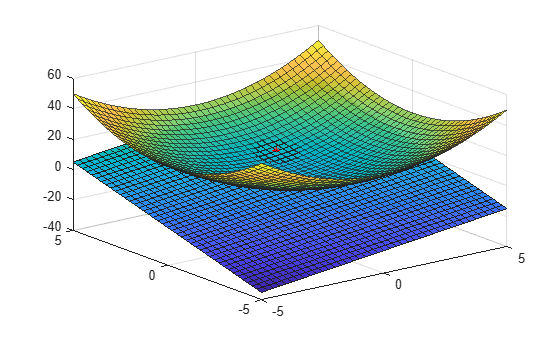## Calculate Tangent Plane to Surface

This example shows how to approximate gradients of a function by finite differences. It then shows how to plot a tangent plane to a point on the surface by using these approximated gradients.

Create the function $f\left(x,y\right)={x}^{2}+{y}^{2}$ using a function handle.

`f = @(x,y) x.^2 + y.^2;`

Approximate the partial derivatives of $f\left(x,y\right)$ with respect to $x$ and $y$ by using the `gradient` function. Choose a finite difference length that is the same as the mesh size.

```[xx,yy] = meshgrid(-5:0.25:5); [fx,fy] = gradient(f(xx,yy),0.25);```

The tangent plane to a point on the surface, $P=\left({x}_{0},{y}_{0},f\left({x}_{0},{y}_{0}\right)\right)$, is given by

`$z=f\left({x}_{0},{y}_{0}\right)+\frac{\partial f\left({x}_{0},{y}_{0}\right)}{\partial x}\left(x-{x}_{0}\right)+\frac{\partial f\left({x}_{0},{y}_{0}\right)}{\partial y}\left(y-{y}_{0}\right).$`

The `fx` and `fy` matrices are approximations to the partial derivatives $\frac{\partial f}{\partial x}$ and $\frac{\partial f}{\partial y}$. The point of interest in this example, where the tangent plane meets the functional surface, is `(x0,y0) = (1,2)`. The function value at this point of interest is `f(1,2) = 5`.

To approximate the tangent plane `z` you need to find the value of the derivatives at the point of interest. Obtain the index of that point, and find the approximate derivatives there.

```x0 = 1; y0 = 2; t = (xx == x0) & (yy == y0); indt = find(t); fx0 = fx(indt); fy0 = fy(indt);```

Create a function handle with the equation of the tangent plane `z`.

`z = @(x,y) f(x0,y0) + fx0*(x-x0) + fy0*(y-y0);`

Plot the original function $f\left(x,y\right)$, the point `P`, and a piece of plane `z` that is tangent to the function at `P`.

```surf(xx,yy,f(xx,yy),'EdgeAlpha',0.7,'FaceAlpha',0.9) hold on surf(xx,yy,z(xx,yy)) plot3(1,2,f(1,2),'r*')```View a side profile.

`view(-135,9)`